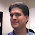Overall Slope Angle vs. Interramp Slope Angle | Mining University

### Overall Slope Angle vs. Interramp Slope Angle

In the mining industry, nomenclature can be very important.  This became especially clear to me a few weeks ago when discussing the slope angle of an open pit mine.  In the context of the discussion, the term 'overall slope angle' was used.  Later, when attempting to apply this 'overall slope angle' I discovered that what we were really talking about was 'interramp slope angle.'

Very often, when professionals in the mining industry say 'slope angle' they are referring to the 'interramp slope angle' or the angle from the toe of one bench to the toe of the next bench, exclusive of any ramp system.  This measure is consistent and easy to visualize.  It includes one bench height, one catch bench and the offset due to the face angle.  This should not be confused with 'overall slope angle' which is the angle between the lowest toe and the highest crest inclusive of any haul roads (Slope Stability in Surface Mining; W.A. Hustrulid p48).

This formal definition of 'overall slope angle' is how we would normally think about the slope of an ordinary hill.  The slope would start at the very top and continue until it flattened out at the bottom.  Over this distance the slope might become more or less steep in a local area but overall it would start at the top and continue to the toe of the hill.  When this same definition is applied to a pit slope in a surface mine, the application of this angle becomes dynamic even if there is no ramp in the slope.

When the formal definition of 'overall slope angle' is applied, the measured angle will change slightly for each additional bench.  The source of this dynamic relationship is the fact that the 'overall slope angle' is equal to the 'interramp slope angle' less one bench width.  For a single bench this difference can be very large.  However, as more and more benches are added the change will become smaller.  Effectively, as the pit becomes deeper the 'overall slope angle' becomes steeper.

A simple way to illustrate this difference is to draw the two slope angles on the same pit starting at the top crest.  The 'overall slope angle' by definition will end at the bottom toe.  The 'interramp slope angle' will touch each crest on the way down but will intersect the pit floor some distance from the bottom toe.  This difference could dramatically affect the interaction of the pit slope and the slope stability defined by the existing rock mechanics.

In mining, as in all other industries, the definitions used can change the outcome of technical discussions.  Please be sure that the terms you are using are the terms that describe what you mean.

1.a diagram would be helpful here.

2.Due to popular request I have re-posted this topic complete with pictures and diagrams. The new post can be found at this link: Overall Slope Angle vs Interramp Slope Angle - Revisited

3.como calculas el overall y el inter-ramp

1.Anonymous,
My Spanish is a little rusty (basically non-existant) but I assume you are asking how to calculate overall vs interramp slope angle.
Overall Slope Angle - The angle formed between the lowest toe and the highest crest as measured from horizontal. Includes the ramp if it passes through the section. Changes as the pit becomes deeper.
Interramp Slope Angle - The angle formed between one crest and the next or one toe and the next as measured from horizontal. Does not include the ramp. Does not change as the pit gets deeper.
For more information please refer to my later post Overall Slope Angle vs Interramp Slope Angle - Revisited
Thanks,
Tony

4.This comment has been removed by a blog administrator.